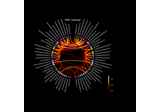# mne.viz.circular_layout#

mne.viz.circular_layout(node_names, node_order, start_pos=90, start_between=True, group_boundaries=None, group_sep=10)[source]#

Create layout arranging nodes on a circle.

Parameters
node_names

Node names.

node_order

List with node names defining the order in which the nodes are arranged. Must have the elements as node_names but the order can be different. The nodes are arranged clockwise starting at “start_pos” degrees.

start_pos`float`

Angle in degrees that defines where the first node is plotted.

start_between`bool`

If True, the layout starts with the position between the nodes. This is the same as adding “180. / len(node_names)” to start_pos.

group_boundaries

List of of boundaries between groups at which point a “group_sep” will be inserted. E.g. “[0, len(node_names) / 2]” will create two groups.

group_sep`float`

Group separation angle in degrees. See “group_boundaries”.

Returns
node_angles`array`, shape=(n_node_names,)

Node angles in degrees.

## Examples using `mne.viz.circular_layout`#Visualize source leakage among labels using a circular graph

Visualize source leakage among labels using a circular graph